RITSEC CTF: CictroHash write-up
Post
Cancel

# RITSEC CTF: CictroHash write-up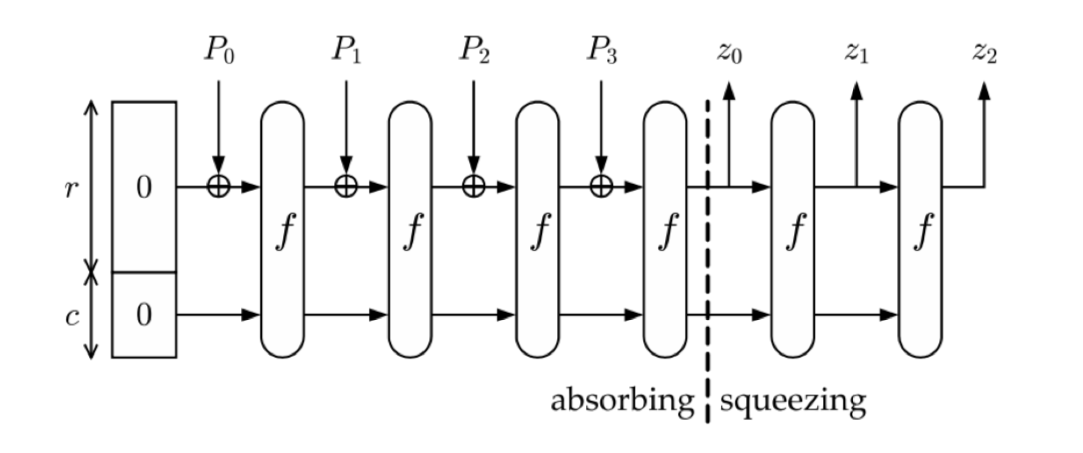# RITSEC CTF: CictroHash write-up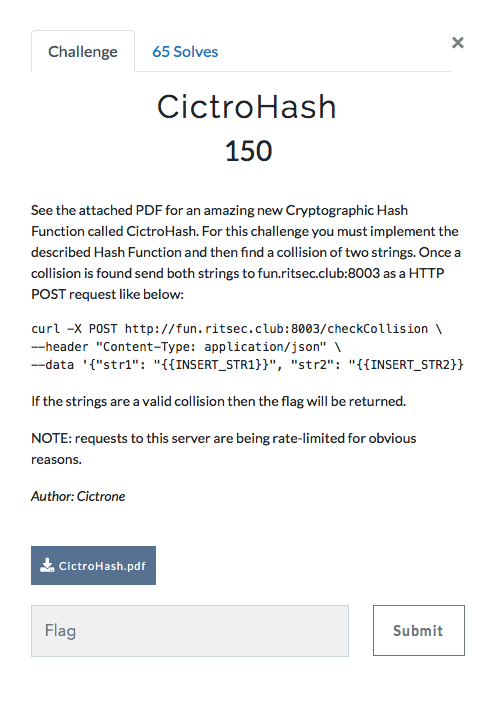After having a look at the attached pdf file we can clearly see that we need to implement a sponge function, which includes XORs and a function f that is comprised of four composite functions. So I’ll start by writing the four functions first.

Visual representation of the sponge functionMathematical definition of f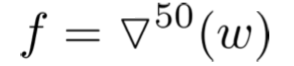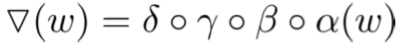## Functions

All of them will have as an argument a matrix of 2x4, as defined in the PDF: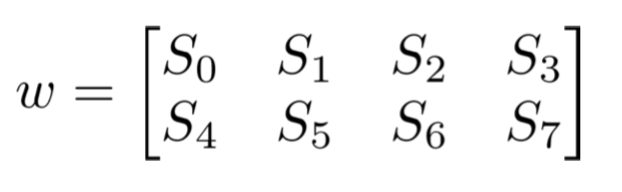I will not be passing the matrix as an argument and then returning it. Instead, I will be modifying it right from within the functions.

### Alpha(w)

The first function was a simple swap, so I just needed to do:

1 2 3 4 5 6 void alpha(unsigned arr){ unsigned x; for (int i = 0; i<4; i++) x[i] = arr[i]; for (int i = 0; i<4; i++) arr[i] = arr[i]; for (int i = 0; i<4; i++) arr[i] = x[i]; } 

### Beta(w)

Beta consisted of XORing one of the elements of the matrix to another:

1 2 3 4 5 void beta(unsigned arr){ for (int i = 0; i<4; i++){ arr[i] ^= arr[3-i]; } } 

### Gamma(w)

In gamma we find that we need to do some variable assignations (we need to take into account that a -> b means store a into b, not the other way around).

1 2 3 4 5 6 7 8 9 10 11 12 13 14 15 void gamma(unsigned arr){ unsigned w,x,y,z; x = arr; y = arr; z = arr; w = arr; arr = arr; arr = arr; arr = arr; arr = z; arr = arr; arr = w; arr = y; arr = x; } 

### Delta(w)

Finally, in delta there are some bitwise rotations that we can do with right and left shifts and masks (to get only the first byte).

1 2 3 4 5 6 7 8 9 10 11 12 13 14 15 16 17 18 unsigned rotate_left(unsigned x){ return 0xff & ((x << 1)|(x >> 7)); } unsigned rotate_right(unsigned x){ return 0xff & ((x >> 1)|(x << 7)); } void delta(unsigned arr){ arr = rotate_left(arr); arr = rotate_left(arr); arr = rotate_left(arr); arr = rotate_left(arr); arr = rotate_right(arr); arr = rotate_right(arr); arr = rotate_right(arr); arr = rotate_right(arr); } 

### The sponge function

After doing the composite function f we need to understand how the sponge function works.It starts with 8 bytes, 4 in r and 4 in c. What we need to do is divide our message in 4-bit chunks (padding it with 0s at the end if needed) and then XOR each chunk with r. Then, we apply f and start again.

For example, if we had “HELLOWORLD” we would divide our message in “HELL”, “OWOR”, “LD” + 00. They would be P0, P1 and P2 respectively.

I transformed this into code the following way:

1 2 3 4 5 6 7 8 9 10 11 12 13 14 15 16 17 18 19 void tests(char stri[], int len){ unsigned w = {31,56,156,167,38,240,174,248}; for (int i=0; i<len/4; i+=1){ for (int j=0; j<4; j++){ w[j] ^= stri[j+(4*i)]; } f(w); } if (len%4!=0){ for (int j=0; j<len%4; j++){ w[j] ^= stri[j+(4*(len/4))]; } for (int j=4-(len%4); j<4; j++){ w[j] ^= 0; } f(w); } printf("0x%x%x%x%x\n", w, w, w, w); } 

## Putting it all together

I ended with the following code, that took 2 arguments when run from the command line: a string and its length. The reason I did this was so that I could pass arguments from another program and then brute-force the solution.

1 2 3 4 5 6 7 8 9 10 11 12 13 14 15 16 17 18 19 20 21 22 23 24 25 26 27 28 29 30 31 32 33 34 35 36 37 38 39 40 41 42 43 44 45 46 47 48 49 50 51 52 53 54 55 56 57 58 59 60 61 62 63 64 65 66 67 68 69 70 71 72 73 74 75 76 77 78 79 80 81 82 83 84 85 86 87 88 #include <stdlib.h> #include <stdio.h> #include <string.h> unsigned rotate_left(unsigned x){ return 0xff & ((x << 1)|(x >> 7)); } unsigned rotate_right(unsigned x){ return 0xff & ((x >> 1)|(x << 7)); } void alpha(unsigned arr){ unsigned x; for (int i = 0; i<4; i++) x[i] = arr[i]; for (int i = 0; i<4; i++) arr[i] = arr[i]; for (int i = 0; i<4; i++) arr[i] = x[i]; } void beta(unsigned arr){ for (int i = 0; i<4; i++){ arr[i] ^= arr[3-i]; } } void gamma(unsigned arr){ unsigned w,x,y,z; x = arr; y = arr; z = arr; w = arr; arr = arr; arr = arr; arr = arr; arr = z; arr = arr; arr = w; arr = y; arr = x; } void delta(unsigned arr){ arr = rotate_left(arr); arr = rotate_left(arr); arr = rotate_left(arr); arr = rotate_left(arr); arr = rotate_right(arr); arr = rotate_right(arr); arr = rotate_right(arr); arr = rotate_right(arr); } void f(unsigned arr){ for (int i=0; i<50; i++){ alpha(arr); beta(arr); gamma(arr); delta(arr); } } void tests(char stri[], int len){ unsigned w = {31,56,156,167,38,240,174,248}; for (int i=0; i<len/4; i+=1){ for (int j=0; j<4; j++){ w[j] ^= stri[j+(4*i)]; } f(w); } if (len%4!=0){ for (int j=0; j<len%4; j++){ w[j] ^= stri[j+(4*(len/4))]; } for (int j=4-(len%4); j<4; j++){ w[j] ^= 0; } f(w); } printf("0x%x%x%x%x\n", w, w, w, w); } int main(int argc, char *argv[]){ int x = strtol(argv, NULL, 10); char stri[x]; strcpy(stri, argv); tests(stri, x); } 

In order to get the flag I wrote a simple python script to generate permutations of letters, pass them as arguments to the C code and then retrieve the hash for comparisons.

1 2 3 4 5 6 7 8 9 10 11 12 13 14 15 16 17 18 19 20 21 22 23 24 25 26 from itertools import product import subprocess, os, sys li = [] def allwords(chars, length): for letters in product(chars, repeat=length): yield ''.join(letters) def main(): letters = "abcdefghijklmnopqrstuvwxyz" global li for wordlen in range(1, 26): for word in allwords(letters, wordlen): print(word) hash = subprocess.Popen(["./Citro", word, str(len(word))], stdout=subprocess.PIPE, stderr=subprocess.PIPE).communicate().strip() print hash if hash in li: print li print word, hash sys.exit() else: li.append(hash) if __name__=="__main__": main() 

After running it for a few seconds we get our output back, a collision was found!

1 2 pa 0xba847264 ap 0xba847264 

Therefore, I do the following Curl request and get the flag:

1 curl -X POST http://fun.ritsec.club:8003/checkCollision --header "Content-Type: application/json" --data '{"str1": "pa", "str2": "ap"}' 

Output

1 2 3 4 { "flag": "RITSEC{I_am_th3_gr3@t3st_h@XOR_3v@}", "success": true }